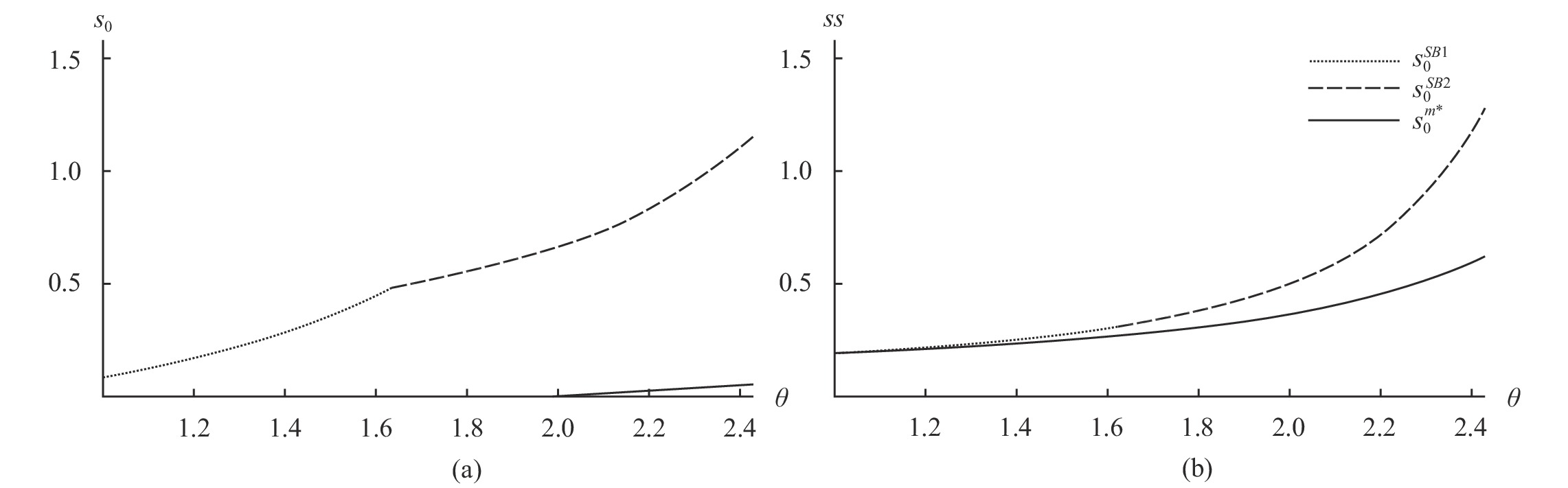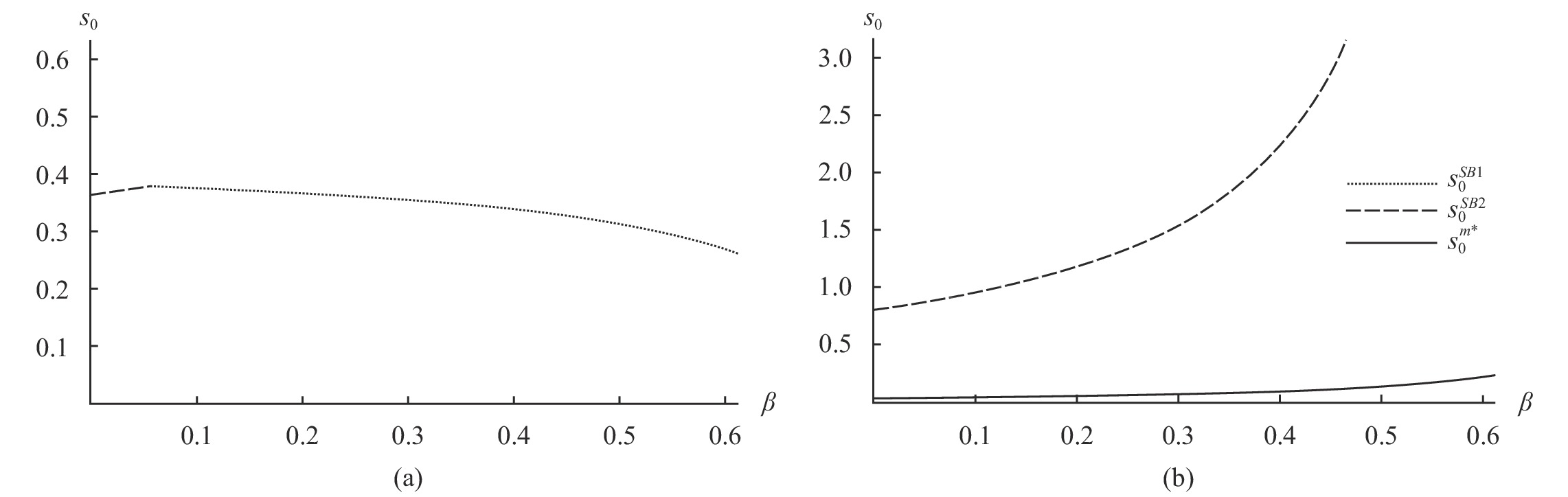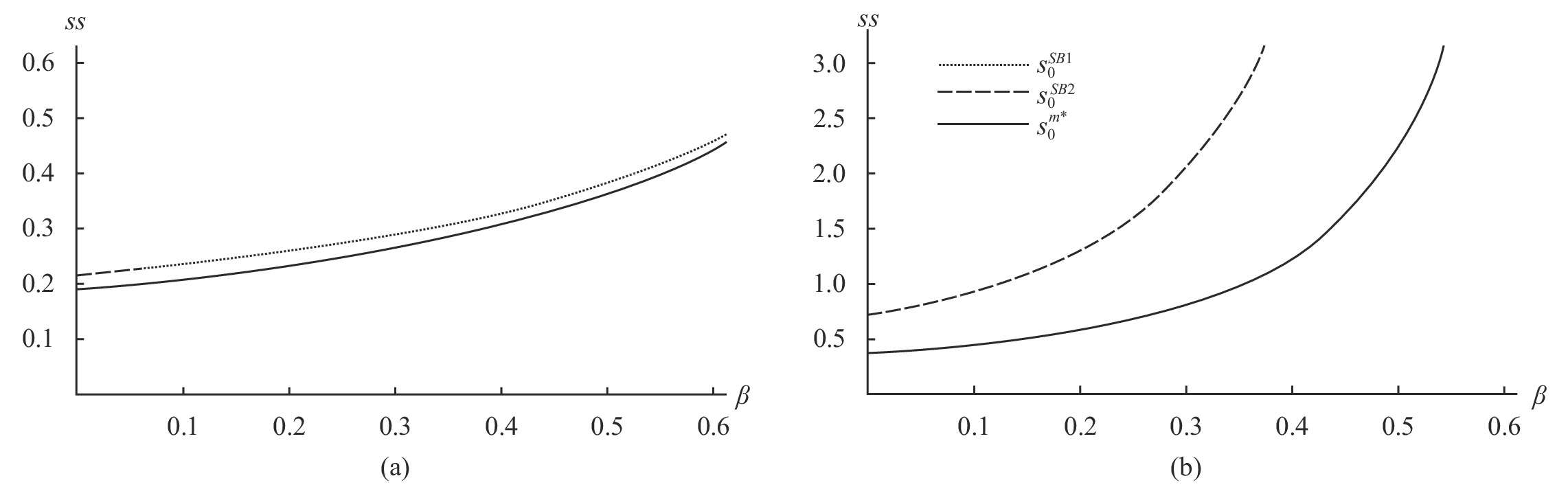﻿ 产品质量、平台治理与政府监管研究《上海财经大学学报》
2021第23卷第6期

 ${Eu}_{ci}=v+[{{na}_{i}}^{e}\left({v}_{a}-p\right)+{{nb}_{i}}^{e}\left({v}_{b}-p\right)]+\beta [{{na}_{i}}^{e}+{{nb}_{i}}^{e}]-{t}_{C}{T}^{i}$ (1)

Hotelling line上 $x$ 处商家的期望利润函数为：

 $E{\pi }_{ai}=(p{{+\alpha )nc}_{i}}^{e}-{p}_{i}-{t}_{F}{T}^{i}$ (2)
 $E{\pi }_{bi}=(p+\alpha ){{nc}_{i}}^{e}-{p}_{i}-{s}_{i}-{t}_{F}{T}^{i}$ (3)

 $E{\pi }_{pli}={p}_{i}\left({{na}_{i}}^{e}+{{nb}_{i}}^{e}\right)-\frac{{{s}_{i}}^{2}}{2}$ (4)

 变量名称 变量定义 $v$ 消费者加入平台获得的效用 ${v}_{a}{\text{、}}{v}_{b}$ $a{\text{、}}b$ 类商家生产的产品带给消费者的额外效用 $\theta$ 度量产品 $a{\text{、}}b$ 之间的质量差异 $p$ 消费者从 $a{\text{、}}b$ 两类商家处购买产品付出的价格 $\beta {\text{、}}\alpha$ 商家（消费者）对消费者（商家）的网络外部性 ${{na}_{i}}^{e}{\text{、}}{{nb}_{i}}^{e}$ 加入平台 $i$ 的 $a{\text{、}}b$ 两类商家的期望数量 ${{nc}_{i}}^{e}$ 加入平台 $i$ 的消费者的期望数量 ${t}_{C}={t}_{F}=t$ 消费者、商家的旅行成本系数 ${p}_{i}$ 平台 $i$ 对商家收取的价格 ${s}_{i}$ 平台 $i$ 的治理强度

 ${{nc}_{0}}^{e}=\frac{1}{2}-\frac{{({nb}_{1}}^{e}-{{nb}_{0}}^{e}+{{na}_{1}}^{e}-{{na}_{0}}^{e}\left)\right({v}_{b}+\beta -p)+\theta ({{na}_{1}}^{e}-{{na}_{0}}^{e})}{2t} , {{nc}_{1}}^{e}=1-{{nc}_{0}}^{e}$ (5)

 ${{na}_{0}}^{e}=\lambda [\frac{1}{2}-\frac{{p}_{0}-{p}_{1}-\left({{nc}_{0}}^{e}-{{nc}_{1}}^{e}\right)\left(p+\alpha \right)}{2t}] , {{na}_{1}}^{e}=\lambda -{{na}_{0}}^{e}$ (6)

 ${{nb}_{0}}^{e}=(1-\lambda )[\frac{1}{2}-\frac{{p}_{0}-{p}_{1}+{s}_{0}-{s}_{1}-\left({{nc}_{0}}^{e}-{{nc}_{1}}^{e}\right)\left(p+\alpha \right)}{2t}], {{nb}_{1}}^{e}=1-\lambda -{{nb}_{0}}^{e}$ (7)

（5）—（7）式说明了平台定价及治理强度对消费者和商家预期的影响。对两类商家而言，拥有更多消费者的平台有更大的吸引力。当 ${p}_{i}$ 越小，吸引的两类商家数量越多， ${s}_{i}$ 越大，吸引的 $b$ 类商家数量越少。对消费者而言，网络外部性 $\beta$ 使得拥有更多商家的平台更具吸引力。除此之外，产品质量也是消费者选择平台的关键考量：高质量产品 $a$ 带来的正的净效用会使得消费者更偏好 $a$ 类商家更多的平台；低质量产品 $b$ 带来的负的净效用会部分抵消网络外部性带来的好处。当 ${v}_{b}-p+\beta > 0$ 时，拥有更多 $b$ 类商家的平台能够吸引更多的消费者，当 ${v}_{b}-p+\beta < 0$ 时，拥有更多 $b$ 类商家的平台会被消费者厌恶。

 ${nc}_{0}=\frac{1}{2}+\frac{\left(1-\lambda \right)\left({s}_{0}-{s}_{1}\right)\left(p-\beta -{v}_{b}\right)+({p}_{0}-{p}_{1})(p-\beta -\lambda \theta -{v}_{b})}{2({t}^{2}-\left(p+\alpha \right)\left(\beta +\lambda {v}_{a}+\left(1-\lambda \right){v}_{b}\right)-p)} \text{，} {nc}_{1}=1-{nc}_{0}$ (8)
 ${na}_{0}=\lambda [\frac{1}{2}+\frac{{t}^{2}\left({p}_{1}-{p}_{0}\right)+\left(p+\alpha \right)\left(1-\lambda \right)\left({s}_{0}-{s}_{1}\right)\left(p-\beta -{v}_{b}\right)}{2\left({t}^{3}+t\left(p+\alpha \right)\left(p-\beta -\lambda \theta -{v}_{b}\right)\right)}] ，{na}_{1}=\lambda -{na}_{0}$ (9)
 ${nb}_{0}=(1-\lambda )[\frac{1}{2}+\frac{{t}^{2}\left({p}_{1}-{p}_{0}+{s}_{1}-{s}_{0}\right)-\left(p+\alpha \right)\lambda \left({s}_{0}-{s}_{1}\right)\left(p-\beta -{v}_{b}-\theta \right)}{2\left({t}^{3}+t\left(p+\alpha \right)\left(p-\beta -\lambda \theta -{v}_{b}\right)\right)}] \text{，} {nb}_{1}=1-\lambda -{nb}_{0}$ (10)

 ${p}_{0}=t+\frac{\left(p+\alpha \right)\left(p-\beta -\lambda \mathrm{\theta }-{v}_{b}\right)}{t}+\frac{\left({s}_{0}-{s}_{1}\right)\left(1-\lambda \right)\left(\left(p+\alpha \right)\lambda \theta -{t}^{2}\right)}{3{t}^{2}}$ (11)
 ${p}_{1}=t+\frac{\left(p+\alpha \right)\left(p-\beta -\lambda \mathrm{\theta }-{v}_{b}\right)}{t}+\frac{\left({s}_{1}-{s}_{0}\right)\left(1-\lambda \right)\left(\left(p+\alpha \right)\lambda \theta -{t}^{2}\right)}{3{t}^{2}}$ (12)

 ${s}_{0}={s}_{1}=\frac{\left(1-\lambda \right)\left(\left(p+\alpha \right)\lambda \theta -{t}^{2}\right)}{3{t}^{2}}$ (13)

 ${s_0}^{c*} = {s_1}^{c*}$ $\dfrac{\left(1-\lambda \right)\left(\left(p+\alpha \right)\lambda \theta -{t}^{2}\right)}{3{t}^{2}}$ ${{p}_{0}}^{c*}={{p}_{1}}^{c*}$ $t+\dfrac{(p+\alpha )(p-\beta -\lambda \mathrm{\theta }-{v}_{b})}{t}$ ${{n}_{a0}}^{c*}={{n}_{a1}}^{c*}$ $\dfrac{\lambda }{2}$ ${{n}_{b0}}^{c*}={{n}_{b1}}^{c*}$ $\dfrac{1-\lambda }{2}$ ${{n}_{c0}}^{c*}={{n}_{c1}}^{c*}$ $\dfrac{1}{2}$

 ${{nc}_{0}}^{e}=\frac{v+{({na}_{0}}^{e}+{{nb}_{0}}^{e}\left)\right({v}_{b}+\beta -p)+{{na}_{0}}^{e}\theta }{t}$ (14)
 ${{na}_{0}}^{e}=\lambda \frac{{{nc}_{0}}^{e}(p+\alpha )-{p}_{0}}{t}$ (15)
 ${{nb}_{0}}^{e}=(1-\lambda )\frac{{{nc}_{0}}^{e}(p+\alpha )-{p}_{0}-{s}_{0}}{t}$ (16)

 ${nc}_{0}=\frac{2v+(1-\beta ){s}_{0}+{p}_{0}(2-2\beta -\theta )}{4-2\beta -\theta } , {na}_{0}=\lambda \frac{2v-2{p}_{0}+(1-\beta ){s}_{0}}{2(4-2\beta -\theta )}, {nb}_{0}=(1-\lambda )\frac{2v-2{p}_{0}+{s}_{0}(\beta +\theta -3)}{2(4-2\beta -\theta )}$ (17)

（17）式反映了平台治理对两边需求的影响：平台治理将提高消费者和 $a$ 类商家的需求；对 $b$ 类商家的影响取决于 $\theta$ ，当 $\theta$ 足够大，即 $3-\beta < \theta < 4-2\beta$ 时，平台治理会提高 $b$ 类商家加入平台的激励。这里平台治理对消费者及 $a$ 类商家的影响符合经济学直觉：平台上低质量产品越少，对消费者吸引力越强，更多的消费者吸引更多 $a$ 类商家加入。对 $b$ 类商家可能产生正面影响的原因在于当 $\theta$ 较大时，平台上 $a$ 类商家数的增多吸引了更多消费者，消费者加入带来的正面影响抵消了平台治理对 $b$ 类商家的负面影响，使得加入平台对 $b$ 类商家仍有利可图。将（17）式代入平台利润函数，令 ${\pi }_{0}$ ${p}_{0}$ 一阶导数等于0，可解得平台设定的价格为：

 ${p}_{0}=\frac{4v-{s}_{0}(2-\theta )}{8}$ (18)

（18）式表明，在 $\theta > 2$ 时，平台治理对产品平均质量的提高有利于提升价格；在 $\theta < 2$ 时，平台治理反而降低了价格。治理对平台定价具有三方面的效应：（1）治理降低了消费者从 $b$ 类商家购买产品的损失，吸引更多消费者进入市场，提高了平台对商家的定价能力；（2）治理减少了 $b$ 类商家数量，降低了商家带来的总外部性，减少了消费者数量，损害了平台对商家的定价能力；（3）治理减少了 $b$ 类商家数量，减少了平台的收入基数。

 ${{s}_{0}}^{m*}=\frac{4v(2-\theta )}{60-32\beta -\theta \left(12+\theta \right)}$ (19)

 ${{s}_{0}}^{m*}$ $\dfrac{4v(\theta -2)}{60-32\beta -12\theta -{\theta }^{2}}$ ${{p}_{0}}^{m*}$ $\dfrac{8v(2\beta +{v}_{a}-4)}{60-32\beta -12\theta -{\theta }^{2}}$ ${{\pi }_{0}}^{m*}$ $\dfrac{8{v}^{2}}{60-32\beta -12\theta -{\theta }^{2}}$ ${{na}_{0}}^{m*}$ $\dfrac{\lambda v(6+\theta )}{60-32\beta -12\theta -{\theta }^{2}}$ ${{nb}_{0}}^{m*}$ $\dfrac{(1-\lambda )v(10-\theta )}{60-32\beta -12\theta -{\theta }^{2}}$ 注：*表示最终均衡结果。

 ${{{s}_{0}}^{c*}-{s}_{0}}^{m*}=\frac{1}{12}(\theta -2)(1-\frac{48v}{60-32\beta -\theta (12+\theta )})$ (20)

$\theta > 2$ 时， ${{s}_{0}}^{m\mathrm{*}}$ ${{s}_{0}}^{c\mathrm{*}}$ 的大小关系取决于（20）式的第三项。为方便分析，令 $v=1/2，$ 通过 ${\theta }^{1}$ ${\theta }^{2}$ $\theta$ 分成两个区间。在 $\theta \epsilon [2,{\theta }^{1}]$ 时：在 $\beta \epsilon[0,\dfrac{(36-12\theta -{\theta }^{2})}{32}]$ 时， ${{{s}_{0}}^{c\mathrm{*}} > {s}_{0}}^{m\mathrm{*}}$ ；在 $\beta \epsilon[\dfrac{\left(36-12\theta -{\theta }^{2}\right)}{32},$ $\dfrac{(38-9\theta -{\theta }^{2})}{24}]$ 时， ${{{s}_{0}}^{c\mathrm{*}} < {s}_{0}}^{m\mathrm{*}}$ 。在 $\theta \epsilon[{\theta }^{1},{\theta }^{2}]\mathrm{时}，{{{s}_{0}}^{c\mathrm{*}} < {s}_{0}}^{m\mathrm{*}}$ 。在寡头市场下，平台竞争将部分抵消产品质量提升带来的优势；而网络外部性的存在，使得将低质量商家推给对手会带来双重损害（自己失去的商家转而加入对手平台）。因此在质量差异较小且网络外部性较弱时，治理对寡头平台带来的损害相对较小，寡头平台的治理强度强于垄断平台。随着质量差异和网络外部性的提高，垄断平台能够完全利用高质量产品的优势，且阻止低质量商家进入对垄断平台的损害更小，此时垄断平台具有更强的治理激励。由此可得出以下命题：

 $cs=\frac{{(2v+\left(1-\beta \right){s}_{0}-{p}_{0}(2-2\beta -\theta \left)\right)}^{2}}{2{(2\beta +\theta -4)}^{2}}$ (21)

 $as=\lambda \frac{{(2v+\left(1-\beta \right){s}_{0}-2{p}_{0})}^{2}}{2{(2\beta +\theta -4)}^{2}},bs=(1-\lambda )\frac{{(2v-2{p}_{0}+{s}_{0}(\beta +\theta -3\left)\right)}^{2}}{2{(2\beta +\theta -4)}^{2}}$ (22)

 $ss=cs+as+bs+{\pi }_{0}$ (23)

 ${{s}_{0}}^{SB}=Min\left\{\frac{4v\left(6-2\beta -\theta \right)\left(8\theta -{\theta }^{2}-4\beta -4\right)}{16\left[25-7\left(4-\beta \right)\beta \right]-16\left[11-\left(9-\beta \right)\beta \right]\theta -4{\left(2-\beta \right)}^{2}{\theta }^{2}+4\left(3-\beta \right){\theta }^{3}-{\theta }^{4}},\frac{4v}{10-3\theta -4\beta }\right\}$ (24)

(一) 质量差异 $\theta$ 对治理强度的影响

$v$ 赋值为 $\dfrac{1}{2}$ $\beta$ 赋值为 $\dfrac{1}{4}$ ，可得平台和政府的治理强度分别为： ${{s}_{0}}^{m\mathrm{*}}=\dfrac{2(\theta -2)}{52-\theta (12+\theta )}$ ${{s}_{0}}^{SB}=$ $Min[\dfrac{2(2\theta -11)(10-15\theta +2{\theta }^{2})}{1180-564\theta -49{\theta }^{2}+44{\theta }^{3}-4{\theta }^{4}},\dfrac{2}{9-3\theta }]$ 图1 $a$ ）展示了 ${{s}_{0}}^{SB}$ ${{s}_{0}}^{m\mathrm{*}}$ $\theta$ 的变化趋势：在 $1 < \theta < 2$ 时，政府希望平台为治理付出一定的努力，但此时平台自身并没有激励进行治理；在 $\theta > 2$ 时，政府的治理强度更高，且二者差距随 $\theta$ 的增加而扩大。图 1 θ对治理强度、社会福利的影响

${{s}_{0}}^{SB}$ ${{s}_{0}}^{m\mathrm{*}}$ 代回，可以得到社会总福利随质量差异 $\theta$ 的变化趋势，如图1 $b$ ）所示：社会总福利随 $\theta$ 的增加而增加，但在 $\theta$ 较小时， ${{s}_{0}}^{SB}$ ${{s}_{0}}^{m\mathrm{*}}$ 下的社会总福利差距十分微小，政府仍可适当放宽对平台的监管力度；随着 $\theta$ 的增加， ${{s}_{0}}^{SB}$ ${{s}_{0}}^{m\mathrm{*}}$ 下社会总福利差距逐渐扩大，政府的监管力度也应随 $\theta$ 的增加而更为严格。最终可得出以下命题：

(二) 网络外部性 $\beta$ 对治理强度的影响图 2 β对治理强度的影响

${{s}_{0}}^{SB}$ ${{s}_{0}}^{m\mathrm{*}}$ 代回，可得出社会总福利随 $\beta$ 变化的趋势，如图3所示。图3 $（a）\mathrm{说}\mathrm{明}$ 在质量差异较小时， ${{s}_{0}}^{SB}$ ${{s}_{0}}^{m\mathrm{*}}$ 下的社会总福利差距十分微小，且差距随 $\beta$ 的增加而减小。此时，政府对平台应当进行宽松的监管，且监管力度应当随 $\beta$ 的增加而减小。图3 $（b）\mathrm{说}\mathrm{明}$ 在质量差异较大时， ${{s}_{0}}^{SB}$ ${{s}_{0}}^{m\mathrm{*}}$ 下的社会总福利差距十分明显，且差距随 $\beta$ 的增加而扩大，此时应密切关注平台的治理强度，且监管力度应当随 $\beta$ 的增加而增加。由此可得出以下命题：图 3 β对社会总福利的影响

 时间 2015-2017 2017-2019 2019-至今 阶段 用户初始积累 平台扩张 体验升级 用户数 1亿（10亿GMV①） 3.44亿（218亿GMV） 6亿（964亿GMV） 发展策略 扩张策略 团购、社交等玩法，拓展基础用户群体 通过补贴、红包、折扣等手段吸引消费者加入 推出品牌特卖专区、百亿补贴等手段 质量策略 基本没有过多措施 下架1070万件疑似侵权；拦截4000万条侵权链接；主动删除的产品是投诉的125倍 97%的疑似侵权链接上线前即被平台封杀；超8.8万家店铺被平台主动关闭 资料来源：拼多多历年《消费者权益报告》、公开招股说明书、年报。

① 过大的 ${ {v}_{a} }$ 将使得消费者市场失去弹性，全部加入平台，因此对 ${\theta }$ 进行限制， ${ \bar{\theta }=3(v-2)+\sqrt{96-80v+9{v}^{2}-32\beta +16v\beta }}$ ；中括号第二项为保证平台利润是价格 ${{p}_{0} }$ 的凹函数。

② 令 ${v=1/2 }$ 是为了保证在 ${ {v}_{a} > 2 }$ 时，加入平台的效用远低于购买产品获得的效用。

${{\theta }^{1}=6\left(\sqrt{2}-1\right)}$ ${{ {\theta }^{2}=\dfrac{1}{2}(\sqrt{233}-9) }}$

④ GMV：Gross Merchandise Volume，代表成交总额，多用于电商行业。

  汪旭晖, 张其林. 平台型网络市场“平台—政府”双元管理范式研究——基于阿里巴巴集团的案例分析[J]. 中国工业经济, 2015, (3).  王勇, 冯骅. 平台经济的双重监管: 私人监管与公共监管[J]. 经济学家, 2017, (11).  王勇, 刘航, 冯骅. 平台市场的公共监管、私人监管与协同监管: 一个对比研究[J]. 经济研究, 2020, (03).  吴德胜, 李维安. 声誉、搜寻成本与网上交易市场均衡[J]. 经济学(季刊), 2008, (4).  谢康, 刘意, 肖静华, 等. 政府支持型自组织构建——基于深圳食品安全社会共治的案例研究[J]. 管理世界, 2017, (8).  Albuquerque P, Pavlidis P, Chatow U. Evaluating promotional activities in an online two-sided market of user-generated content[J].Marketing Science,2012,31(3):406–432.  Amelio A, Jullien B. Tying and freebies in two-sided markets[J].International Journal of Industrial Organization,2012,30(5):436–446.  Anderson S P, Peitz M. Media see-saws: Winners and losers in platform markets[J].Journal of Economic Theory,2020,186:1–43.  Armstrong M. Competition in two-sided markets[J].The RAND Journal of Economics,2006,37(3):668–691.  Armstrong M, Wright J. Two-sided markets, competitive bottlenecks and exclusive contracts[J].Economic Theory,2007,32(2):353–380.  Belleflamme P, Peitz M. Managing competition on a two-sided platform[J]. Journal Economics & Management Strategy,2019,28(1):5–22.  Belleflamme P, Toulemonde E. Negative intra-group externalities in two-sided markets[J].International Economic Review,2009,50(1):245–272.  Brühn T, Georg G. Exclusionary practices in two-sided markets: The effect of radius clauses on competition between shopping centers[J].Managerial and Decision Economics,2018,39(5):577–590.  Caillaud B, Jullien B. Chicken & egg: Competition among intermediation service providers[J].The RAND Journal of Economics,2003,34(2):309–328.  Economides N, Tag J. Network neutrality on the internet: A two-sided market analysis[J]. Information Economics & Policy,2012,24(2):91–104.  Farrell J, Katz M L. Innovation, rent extraction, and integration in systems markets[J]. Journal of Industrial Economics,2000,48(4):413–432.  Gawer A, Cusumano M A. Platform leadership: How intel, microsoft, and cisco drive industry innovation[M]. Harvard Business School Press, 2002.  Hagiu A, Lee R S. Exclusivity and control[J].Journal of Economics & Management Strategy,2011,20(3):679–708.  Hu D, Zhao J L, Cheng J. Reputation management in an open source developer social network: An empirical study on determinants of positive evaluations[J].Decision Support Systems,2012,53(3):526–533.  Iansiti M, Levien R. The keystone advantage: What the new dynamics of business ecosystems mean for strategy, innovation, and sustainability[M]. Harvard Business Press, 2004.  Jiang Y, Guo H. Design of consumer review systems and product pricing[J].Information Systems Research,2015,26(4):714–730.  Liu Q, Serfes K. Price discrimination in two-sided markets[J]. Journal of Economics & Management Strategy,2013,22(4):768–786.  Luca M, Zervas G. Fake it till you make it: Reputation, competition, and yelp review fraud[J].Management Science,2016,62(12):3412–3427.  Rochet J, Tirole J. Platform competition in two-sided markets[J].Journal of the European Economic Association,2003,1(4):990–1029.  Rochet J, Tirole J. Two-sided markets: A progress report[J].Rand Journal of Economics,2006,37(3):645–667.  Schuckert M, Liu X, Law R. Stars, votes, and badges: How online badges affect hotel reviewers[J]. Journal of Travel & Tourism Marketing,2016,33(4):440–452.
Research on Product Quality, Platform Governance and Government Supervision
Zou Gan
School of Business, Shanghai University of Finance and Economics, Shanghai 200433, China
Abstract: As an increasingly important form of industrial organization in the new economic era, the platform economy has become an important engine for promoting economic transformation and development. With the development of the platform economy, the monopoly power of platform companies has become stronger and stronger, and more and more problems in the operation of internet platforms have been exposed. What follows is the corporate governance of platforms and the government governance of platform enterprises. The governance intensity of low-quality business by platforms is often lower than the optimal level in society, resulting in damage to consumer rights and social welfare. To clarify the selection incentives and impacts of platform governance strategies, based on the social reality of platform sales of counterfeit products, in the situation where there are both high-quality and low-quality merchants, this paper compares the governance strength of platforms in the oligopoly market and the monopoly market respectively by constructing a game model, and further analyzes the regulatory strategies in different market environments from the perspective of government supervision.By comparing the equilibrium results under different market structures and governance models, this paper finds that the quality difference between the two types of products is the key to determining the governance intensity of platforms. The greater the quality difference, the stronger the platform governance intensity. The difference in the governance intensity of platforms under different market structures depends on network externality and product quality differences: The stronger the network externality and the greater the quality difference, the stronger the governance intensity under the monopoly market; the weaker the network externality and the smaller the quality difference, the stronger the governance intensity under the oligopolistic market. This paper finally analyzes how to regulate platform governance from the perspective of government supervision. Compared with the social optimal, the strength of platform governance is weak, and the increase in network externality and quality differences will further enlarge the gap between the two. Therefore, the intensity of government supervision should be determined according to the market environment, increasing with the increase of network externality and product quality differences.This paper enriches the theoretical exploration of platform economy from the perspective of business governance, and meticulously describes the relationship between governance intensity and network externality as well as business quality. The research conclusions have a certain enlightening effect on the choice of platform self-governance strategies, the choice of government governance intensity, the protection of consumer rights and the promotion of social welfare.
Key words: platform economy    platform governance    government supervision    social welfare